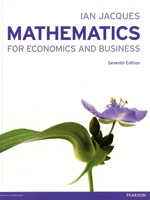Mathematics for Economics & Business, 7th edition

By Ian Jacques
December 2012
Pearson Education
Distributed by Trans-Atlantic Publications
ISBN: 9780273763567325 pages, Illustrated
\$57.50 Paper Original

With its friendly and informal style, this market leading text breaks down topics into short sections making learning each new technique seem less daunting. With plenty of practice problems, it provides opportunities to stop and check understanding and allows students to learn at their own pace.

Contents:

Preface
Guided tour of the textbook
Guided tour of the online resources

Introduction: Getting Started
Notes for students: how to use this book
CHAPTER 1 Linear Equations
1.1 Introduction to algebra
1.2 Further algebra
1.3 Graphs of linear equations
1.4 Algebraic solution of simultaneous linear equations
1.5 Supply and demand analysis
1.6 Transposition of formulae
1.7 National income determination

Formal mathematics

CHAPTER 2 Non-linear Equations
2.2 Revenue, cost and profit
2.3 Indices and logarithms
2.4 The exponential and natural logarithm functions

Formal mathematics

CHAPTER 3 Mathematics of Finance
3.1 Percentages
3.2 Compound interest
3.3 Geometric series
3.4 Investment appraisal

Formal mathematics

CHAPTER 4 Differentiation
4.1 The derivative of a function
4.2 Rules of differentiation
4.3 Marginal functions
4.4 Further rules of differentiation
4.5 Elasticity
4.6 Optimisation of economic functions
4.7 Further optimisation of economic functions
4.8 The derivative of the exponential and natural logarithm functions

Formal mathematics

CHAPTER 5 Partial Differentiation
5.1 Functions of several variables
5.2 Partial elasticity and marginal functions
5.3 Comparative statics
5.4 Unconstrained optimization
5.5 Constrained optimization
5.6 Lagrange multipliers

Formal mathematics

CHAPTER 6 Integration
6.1 Indefinite integration
6.2 Definite integration

Formal mathematics

CHAPTER 7 Matrices
7.1 Basic matrix operations
7.2 Matrix inversion
7.3 Cramer's rule

Formal mathematics

CHAPTER 8 Linear Programming
8.1 Graphical solution of linear programming problems
8.2 Applications of linear programming

Formal mathematics

CHAPTER 9 Dynamics
9.1 Difference equations
9.2 Differential equations

Formal mathematics

Glossary
Index

The following additional material is available as part of the online resources supporting this title either at www.pearsoned.co.uk/jacques or included in MyMathLab.

Solutions to Problems
Excel
Maple
AT1 Differentiation from First Principles
AT2 Implicit Differentiation
AT3 Hessians
AT4 Input-Output Analysis